# Interpret all statistics for Store Descriptive Statistics

Find definitions and interpretation guidance for every statistic that is provided with store descriptive statistics.

## Mean

The mean is the average of the data, which is the sum of all the observations divided by the number of observations.

For example, the wait times (in minutes) of five customers in a bank are: 3, 2, 4, 1, and 2. The mean waiting time is calculated as follows: On average, a customer waits 2.4 minutes for service at the bank.

### Interpretation

Use the mean to describe the sample with a single value that represents the center of the data. Many statistical analyses use the mean as a standard measure of the center of the distribution of the data.

The median and the mean both measure central tendency. But unusual values, called outliers, can affect the median less than they affect the mean. If your data are symmetric, the mean and median are similar.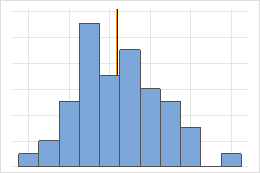For the symmetric distribution, the mean (blue line) and median (orange line) are so similar that you can't easily see both lines. But the non-symmetric distribution is skewed to the right.

## SE mean

The standard error of the mean (SE Mean) estimates the variability between sample means that you would obtain if you took repeated samples from the same population. Whereas the standard error of the mean estimates the variability between samples, the standard deviation measures the variability within a single sample.

For example, you have a mean delivery time of 3.80 days, with a standard deviation of 1.43 days, from a random sample of 312 delivery times. These numbers yield a standard error of the mean of 0.08 days (1.43 divided by the square root of 312). If you took multiple random samples of the same size, from the same population, the standard deviation of those different sample means would be around 0.08 days.

### Interpretation

Use the standard error of the mean to determine how precisely the sample mean estimates the population mean.

A smaller value of the standard error of the mean indicates a more precise estimate of the population mean. Usually, a larger standard deviation results in a larger standard error of the mean and a less precise estimate of the population mean. A larger sample size results in a smaller standard error of the mean and a more precise estimate of the population mean.

Minitab uses the standard error of the mean to calculate the confidence interval.

## StDev

The standard deviation is the most common measure of dispersion, or how spread out the data are about the mean. The symbol σ (sigma) is often used to represent the standard deviation of a population, while s is used to represent the standard deviation of a sample. Variation that is random or natural to a process is often referred to as noise.

Because the standard deviation is in the same units as the data, it is usually easier to interpret than the variance.

### Interpretation

Use the standard deviation to determine how spread out the data are from the mean. A higher standard deviation value indicates greater spread in the data. A good rule of thumb for a normal distribution is that approximately 68% of the values fall within one standard deviation of the mean, 95% of the values fall within two standard deviations, and 99.7% of the values fall within three standard deviations.

The standard deviation can also be used to establish a benchmark for estimating the overall variation of a process.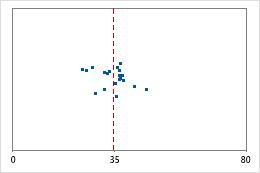Hospital discharge timesAdministrators track the discharge time for patients who are treated in the emergency departments of two hospitals. Although the average discharge times are about the same (35 minutes), the standard deviations are significantly different. The standard deviation for hospital 1 is about 6. On average, a patient's discharge time deviates from the mean (dashed line) by about 6 minutes. The standard deviation for hospital 2 is about 20. On average, a patient's discharge time deviates from the mean (dashed line) by about 20 minutes.

## Variance

The variance measures how spread out the data are about their mean. The variance is equal to the standard deviation squared.

### Interpretation

The greater the variance, the greater the spread in the data.

Because variance (σ2) is a squared quantity, its units are also squared, which may make the variance difficult to use in practice. The standard deviation is usually easier to interpret because it's in the same units as the data. For example, a sample of waiting times at a bus stop may have a mean of 15 minutes and a variance of 9 minutes2. Because the variance is not in the same units as the data, the variance is often displayed with its square root, the standard deviation. A variance of 9 minutes2 is equivalent to a standard deviation of 3 minutes.

## CVariation

The coefficient of variation (denoted as COV) is a measure of spread that describes the variation in the data relative to the mean. The coefficient of variation is adjusted so that the values are on a unitless scale. Because of this adjustment, you can use the coefficient of variation instead of the standard deviation to compare the variation in data that have different units or that have very different means.

### Interpretation

The larger the coefficient of variation, the greater the spread in the data.

For example, you are the quality control inspector at a milk bottling plant that bottles small and large containers of milk. You take a sample of each product and observe that the mean volume of the small containers is 1 cup with a standard deviation of 0.08 cup, and the mean volume of the large containers is 1 gallon (16 cups) with a standard deviation of 0.4 cups. Although the standard deviation of the gallon container is five times greater than the standard deviation of the small container, their coefficients of variation support a different conclusion.
Large container Small container
COV = 100 * 0.4 cups / 16 cups = 2.5 COV = 100 * 0.08 cups / 1 cup = 8
The coefficient of variation of the small container is more than three times greater than that of the large container. In other words, although the large container has a greater standard deviation, the small container has much more variability relative to its mean.

## Q1

Quartiles are the three values–the first quartile at 25% (Q1), the second quartile at 50% (Q2 or median), and the third quartile at 75% (Q3)–that divide a sample of ordered data into four equal parts.

The first quartile is the 25th percentile and indicates that 25% of the data are less than or equal to this value.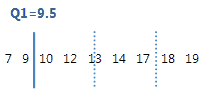For this ordered data, the first quartile (Q1) is 9.5. That is, 25% of the data are less than or equal to 9.5.

## Median

The median is the midpoint of the data set. This midpoint value is the point at which half the observations are above the value and half the observations are below the value. The median is determined by ranking the observations and finding the observation that are at the number [N + 1] / 2 in the ranked order. If the number of observations are even, then the median is the average value of the observations that are ranked at numbers N / 2 and [N / 2] + 1.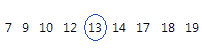For this ordered data, the median is 13. That is, half the values are less than or equal to 13, and half the values are greater than or equal to 13. If you add another observation equal to 20, the median is 13.5, which is the average between 5th observation (13) and the 6th observation (14).

### Interpretation

The median and the mean both measure central tendency. But unusual values, called outliers, can affect the median less than they affect the mean. If your data are symmetric, the mean and median are similar.For the symmetric distribution, the mean (blue line) and median (orange line) are so similar that you can't easily see both lines. But the non-symmetric distribution is skewed to the right.

## Q3

Quartiles are the three values–the first quartile at 25% (Q1), the second quartile at 50% (Q2 or median), and the third quartile at 75% (Q3)–that divide a sample of ordered data into four equal parts.

The third quartile is the 75th percentile and indicates that 75% of the data are less than or equal to this value.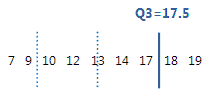For this ordered data, the third quartile (Q3) is 17.5. That is, 75% of the data are less than or equal to 17.5.

## IQR

The interquartile range (IQR) is the distance between the first quartile (Q1) and the third quartile (Q3). 50% of the data are within this range.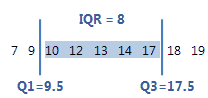For this ordered data, the interquartile range is 8 (17.5–9.5 = 8). That is, the middle 50% of the data is between 9.5 and 17.5.

### Interpretation

Use the interquartile range to describe the spread of the data. As the spread of the data increases, the IQR becomes larger.

## TrMean

The mean of the data, without the highest 5% and lowest 5% of the values.

Use the trimmed mean to eliminate the impact of very large or very small values on the mean. When the data contain outliers, the trimmed mean may be a better measure of central tendency than the mean.

## Sum

The sum is the total of all the data values. The sum is also used in statistical calculations, such as the mean and standard deviation.

## Minimum

The minimum is the smallest data value.

In these data, the minimum is 7.

 13 17 18 19 12 10 7 9 14

### Interpretation

Use the minimum to identify a possible outlier or a data-entry error. One of the simplest ways to assess the spread of your data is to compare the minimum and maximum. If the minimum value is very low, even when you consider the center, the spread, and the shape of the data, investigate the cause of the extreme value.

## Maximum

The maximum is the largest data value.

In these data, the maximum is 19.

 13 17 18 19 12 10 7 9 14

### Interpretation

Use the maximum to identify a possible outlier or a data-entry error. One of the simplest ways to assess the spread of your data is to compare the minimum and maximum. If the maximum value is very high, even when you consider the center, the spread, and the shape of the data, investigate the cause of the extreme value.

## Range

The range is the difference between the largest and smallest data values in the sample. The range represents the interval that contains all the data values.

### Interpretation

Use the range to understand the amount of dispersion in the data. A large range value indicates greater dispersion in the data. A small range value indicates that there is less dispersion in the data. Because the range is calculated using only two data values, it is more useful with small data sets.

## SSQ

The uncorrected sum of squares is the sum of the squares of each value in the column. For example, if the column contains x1, x2, ... , xn, then sum of squares calculates (x12 + x22 + ... + xn2). Unlike the corrected sum of squares, the uncorrected sum of squares includes error. The data values are squared without first subtracting the mean.

## Skewness

Skewness is the extent to which the data are not symmetrical.

### Interpretation

Use skewness to help you establish an initial understanding of your data.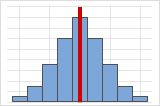Symmetrical or non-skewed distributionsAs data becomes more symmetrical, its skewness value approaches zero. Figure A shows normally distributed data, which by definition exhibits relatively little skewness. By drawing a line down the middle of this histogram of normal data it's easy to see that the two sides mirror one another. But lack of skewness alone doesn't imply normality. Figure B shows a distribution where the two sides still mirror one another, though the data is far from normally distributed.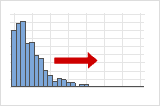Positive or right skewed distributionsPositive skewed or right skewed data is so named because the "tail" of the distribution points to the right, and because its skewness value will be greater than 0 (or positive). Salary data is often skewed in this manner: many employees in a company make relatively little, while increasingly few people make very high salaries.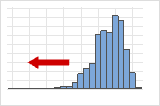Negative or left skewed distributionsLeft skewed or negative skewed data is so named because the "tail" of the distribution points to the left, and because it produces a negative skewness value. Failure rate data is often left skewed. Consider light bulbs: very few will burn out right away, the vast majority lasting for quite a long time.

## Kurtosis

Kurtosis indicates how the tails of a distribution differ from the normal distribution.

### Interpretation

Use kurtosis to initially understand general characteristics about the distribution of your data.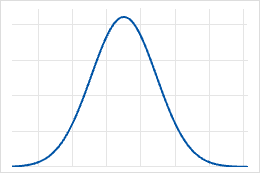Baseline: Kurtosis value of 0Normally distributed data establish the baseline for kurtosis. A kurtosis value of 0 indicates that the data follow the normal distribution perfectly. A kurtosis value that significantly deviates from 0 may indicate that the data are not normally distributed.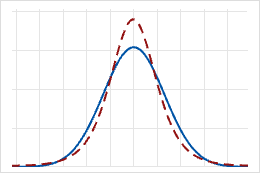Positive kurtosisA distribution that has a positive kurtosis value indicates that the distribution has heavier tails than the normal distribution. For example, data that follow a t-distribution have a positive kurtosis value. The solid line shows the normal distribution, and the dotted line shows a distribution that has a positive kurtosis value.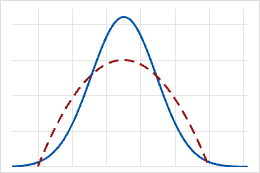Negative kurtosisA distribution with a negative kurtosis value indicates that the distribution has lighter tails than the normal distribution. For example, data that follow a beta distribution with first and second shape parameters equal to 2 have a negative kurtosis value. The solid line shows the normal distribution and the dotted line shows a distribution that has a negative kurtosis value.

## MSSD

The MSSD is the mean of the squared successive difference. MSSD is an estimate of variance. One possible use of the MSSD is to test whether a sequence of observations is random. In quality control, a possible use of MSSD is to estimate the variance when the subgroup size = 1.

## N

The number of non-missing values in the sample.

In this example, there are 141 recorded observations.
Total count N N*
149 141 8

## NMissing

The number of missing values in the sample. The number of missing values refers to cells that contain the missing value symbol *.

In this example, 8 errors occurred during data collection and are recorded as missing values.
Total count N NMissing
149 141 8

## Count

The total number of observations in the column. Use to represent the sum of N missing and N nonmissing.

In this example, there are 141 valid observations and 8 missing values. The count is 149.
Count N NMissing
149 141 8

## CumN

Cumulative N is a running total of the number of observations in successive categories. For example, an elementary school records the number of students in grades one through six. The CumN column contains the cumulative count of the student population:
Grade Level Count CumN Calculation
1 49 49 49
2 58 107 49 + 58
3 52 159 49 + 58 + 52
4 60 219 49 + 58 + 52 + 60
5 48 267 49 + 58 + 52 + 60 + 48
6 55 322 49 + 58 + 52 + 60 + 48 + 55

## Percent

The percent of observations in each group of the By variable. In the following example, there are four groups: Line 1, Line 2, Line 3, and Line 4.

Group (by variable) Percent
Line 1 16
Line 2 20
Line 3 36
Line 4 28

## CumP

The cumulative percent is the cumulative sum of the percentages for each group of the By variable. In the following example, the By variable has 4 groups: Line 1, Line 2, Line 3, and Line 4.

Group (by variable) Percent CumP
Line 1 16 16
Line 2 20 36
Line 3 36 72
Line 4 28 100
By using this site you agree to the use of cookies for analytics and personalized content.  Read our policy